## INTRODUCTION

Soon after Harry Markowitz published his landmark 1952 article on portfolio selection, the correlation coefficient assumed vital significance as a measure of diversification and an input to portfolio construction. More recently, investors have come to recognize the importance of correlation to a wide variety of investment activities. Analysts have used the parameter to detect regime shifts, describe markets as ‘risk-on/risk-off’ and justify the underperformance of stock pickers. Of course, to monitor the tangled web of relationships between assets can be daunting. To cover a universe of 10 assets, one must track 45 pair-wise correlations. Kritzman and Li (2010) introduced what is perhaps the first measure to capture the degree of correlation ‘unusualness’ across a set of assets through time. Their financial turbulence score spikes when asset prices ‘behave in an uncharacteristic fashion, including extreme price moves, decoupling of correlated assets, and convergence of uncorrelated assets’. For at least two reasons, this framework is well suited to the purpose of quantifying correlation surprises. First, it summarizes in a single measure the collective unusualness of correlations across any universe of assets. Second, rather than identify whether correlations are high or low, it measures the degree to which interactions depart from their historical norms, whatever those may be.

In this article, we extend Kritzman and Li’s study by disentangling the volatility and correlation components of turbulence to derive a measure of correlation surprise. We also show how correlation surprise is orthogonal to volatility and present empirical evidence that it contains incremental forward-looking information. On average, after controlling for volatility, we find that periods characterized by correlation surprise lead to higher risk and lower returns to risk premia than periods characterized by typical correlations. This result holds across many markets including US equities, European equities and foreign exchange. Our results corroborate the predictive capacity of turbulence and suggest that its decomposition may also prove fruitful in forecasting investment performance.

This article is organized as follows. First, we review the methodology behind Kritzman and Li’s financial turbulence measure, also known as the Mahalanobis distance, and review its empirical features. Next, we show how to decompose turbulence to isolate the contribution of correlation surprises. We then present empirical evidence that correlation surprises contain incremental information about future risk and return at both daily and monthly frequencies.

## THE MAHALANOBIS DISTANCE AS A MEASURE OF FINANCIAL TURBULENCE

Using a formula originally developed by Mahalanobis (1927, 1936) to categorize human skulls and later employed by Chow et al (1999) to stress test portfolios for turbulent periods, Kritzman and Li (2010) define their financial turbulence statistic as a multivariate unusualness score. We adopt the same definition of turbulence but simply divide by the number of assets (which is constant through time) in order to facilitate ease of interpretation in subsequent sections of this article: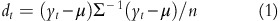where

d t :

turbulence for a particular time period t (scalar)

y t :

vector of asset returns for period t (1 × n vector)

μ :

sample average vector of historical returns (1 × n vector)

Σ:

sample covariance matrix of historical returns (n × n matrix)

n :

number of assets in universe

This statistic, which can be thought of as a multivariate z-score, measures the statistical unusualness of a contemporaneous cross-section of asset returns relative to its historical distribution. It captures the extent to which the risk-adjusted magnitudes of the returns differ from their historical means as well as the extent to which their interaction is inconsistent with the historical correlation matrix. Turbulence is different from cross-sectional volatility, which measures the dispersion around the cross-sectional mean but ignores the time series means.Footnote 1 It is also different from the rolling volatility of a portfolio because it describes the unusualness of a particular day rather than the dispersion in returns over a period of time.

Empirically, turbulence has several attractive features:

• It tends to spike during recognizable periods of market stress that are characterized by heightened volatility and correlation breakdowns.

• It is linked to investment performance; on average, returns to a wide variety risk premia are significantly lower during turbulent periods.Footnote 2

• It is persistent. Turbulent episodes tend to cluster in time and do not subside immediately after they arise.

Taken together, the persistence of turbulence and its link with returns suggest that investors could enhance performance by de-risking when turbulence first strikes. For example, Kritzman and Li show how to improve the performance of a foreign exchange carry strategy by reducing exposure when currency turbulence rises. In this article, we put the persistence of turbulence – and its relationship with subsequent return and risk – under the microscope by disentangling correlation surprises from magnitude surprises.

## ISOLATING CORRELATION SURPRISES

The turbulence methodology is ideally suited to detecting periods when the co-movement between assets differs from what we would expect based on historical correlations. For a given day, the turbulence score captures both the average degree of unusualness in individual asset returns (magnitude surprise) and the degree of unusualness in the interaction between each pair of assets (correlation surprise). To disentangle these two components and isolate the degree of correlation surprise, we first compute the magnitude surprise. Magnitude surprise is equal to the turbulence score, given in equation (1), where all off-diagonal elements in the covariance matrix are set to zero.Footnote 3 This ‘correlation-blind’ turbulence measure captures magnitude surprises, but ignores whether co-movement is typical or atypical. Next, we divide the standard turbulence score – which includes correlation effects – by the magnitude surprise. This ratio is the correlation surprise: the unusualness of interactions on a particular day relative to history.

A correlation surprise ratio greater than one is associated with correlation breakdowns (that is, previously correlated assets diverging and/or previously negatively correlated assets converging). A correlation surprise ratio less than one is associated with relatively typical correlation outcomes. In other words, days characterized by low correlation surprise are actually less unusual than the magnitudes of the individual returns alone would suggest. To review, we compute the following quantities to calculate correlation surprise:

1. 1

Magnitude surprise: a ‘correlation-blind’ turbulence score in which all off-diagonals in the covariance matrix are set to zero.

2. 2

Turbulence score: the degree of statistical unusualness across assets on a given day, as given in equation (1).

3. 3

Correlation surprise: the ratio of turbulence to magnitude surprise, using the above quantities (2) and (1), respectively.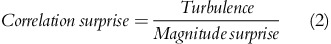To build intuition around the turbulence and correlation surprise scores, let us start by considering a single asset, x. In this case, turbulence is simply equal to the squared z-score of the asset return, as shown in equation (3). To simplify the formulas in this section, we will assume (without loss of generality) that the average value of all relevant return streams are zero, and we will simply denote the asset's current period return observation as x.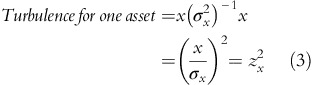It is impossible, by definition, for one asset to exhibit any correlation surprise. If we consider two assets, we can think of turbulence as a multivariate z-score. Rather than normalizing only by the variance of an asset, as in equation (3), turbulence now normalizes for the entire covariance matrix of the assets, which accounts for their historical variances and the correlation between them as shown in equation (4).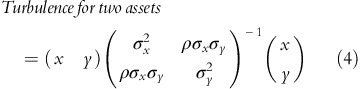By dividing turbulence by magnitude surprise, we can express the correlation surprise for two assets as shown in equation (5) (the derivation of this formula is provided in the Appendix). We see that correlation surprise is expressed in terms of normalized asset z-scores and the historical correlation, ρ. All units of magnitude cancel out in this formula.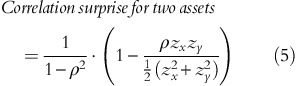Turbulence and correlation surprise also have intuitive geometric interpretations. Consider a simple empirical example in which our universe consists of two assets, A and B, with means of zero, volatilities of 5 per cent, and a correlation of 0.5. Figure 1 shows the turbulence score, magnitude surprise and correlation surprise for two multivariate return observations.

The dashed ellipse in Figure 1 is the iso-turbulence ellipse. All observations that fall along this ellipse have the same turbulence score. Its slant reflects the positive correlation between the two assets: in any given period, it is more likely that A and B move in the same direction than in opposite directions. In other words, when A and B move in the same direction, we require a larger return magnitude to produce the same degree of turbulence. In this example, period 2 is more turbulent than period 1 despite the fact that the magnitudes of the two observations are identical (each has a magnitude surprise of 1.0). Period 2 has a higher correlation surprise than period 1 because it reflects an outcome where the two assets, which are expected to move together, diverge.

The correlation surprise score is akin to a compass: it contains information about the multivariate direction along which the observation falls. It does not measure the magnitude of returns. The diagonal lines in Figure 2 are iso-correlation surprise vectors; regardless of their magnitude, any two points along one of these lines will have the same correlation surprise score.

## HOW DOES CORRELATION SURPRISE DIFFER FROM OTHER MEASURES THAT INCORPORATE CORRELATION?

To our knowledge, correlation surprise is the only measure that isolates the degree to which the co-movement across a set of assets is typical or atypical relative to history.Footnote 4 Below we list three other measures that are sensitive to correlation shifts and describe the important ways in which they fail to capture correlation surprises.

• Rolling correlation: Investors sometimes monitor the rolling correlation between two assets to help them identify regime shifts. Whereas rolling correlation is a pair-wise measure, correlation surprise can capture in a single parameter the unusualness of co-movement across a large universe of assets.Footnote 5 Indeed, the number of pair-wise correlations quickly becomes unmanageable as the asset universe expands. Again, to monitor a 10-asset universe, we would be required to estimate 45 rolling correlations; for a 100-asset universe, the number increases to 4950.Footnote 6

• Cross-sectional volatility: Cross-sectional volatility is different from correlation surprise because it fails to account for the degree to which a particular correlation outcome is typical or atypical. Put differently, cross-sectional volatility spikes when asset returns diverge, regardless of whether their typical correlation is 10 per cent or 90 per cent. All else equal, a divergence is far more unusual in the latter case than in the former.

• Multivariate Generalized Autoregressive Conditional Heteroskedasticity (GARCH) models: This class of models extends the univariate GARCH framework to derive volatility forecasts based on lagged covariance terms as well as lagged variance terms. For example, a multivariate GARCH model might forecast the volatility of a two-stock portfolio based on lagged innovations in stock A, lagged innovations in stock B and lagged covariance terms between stocks A and B.Footnote 7 Engle (2002) has proposed a Dynamic Conditional Correlation model, which is a simple class of multivariate GARCH models. However, to our knowledge, none of the myriad multivariate GARCH specifications account for interactions between variance and covariance terms.Footnote 8 And, as we will demonstrate in the next section, volatile episodes characterized by atypical correlations tend to be more persistent and severe than volatile episodes characterized by typical correlations. The decomposition of the Mahalanobis distance enables us to analyze the intertemporal relationship between correlation and magnitude surprises in a way that multivariate GARCH models do not.

## DATA AND RESULTS

We construct correlation surprise series for three asset universes: US equities, European equities and currencies. Table 1 provides details regarding the component indices, start date and lookback window for each series.

Figure 3 shows a scatter-plot of daily correlation surprise versus magnitude surprise scores for each asset universe. For the purposes of this figure, we converted each metric into a per cent rank with regard to its full sample distribution. The dark lines show the best fit linear regression line for each plot.

It is apparent from visual inspection of Figure 3 that the correlation and magnitude surprise scores capture different information (which is collectively captured in the turbulence score). In fact, on a contemporaneous basis the two metrics are negatively correlated. What is intriguing is that despite this contemporaneous negative correlation, we find strong empirical evidence that high correlation surprise tends to precede higher risk and also lower returns. We will discuss these findings in more detail shortly. Before we shift our focus to empirics, let us first consider a more theoretical question: why would we expect unusual correlations to precede heightened volatility and lower returns? There are several plausible reasons. Investors who build correlation assumptions into their models – either explicitly or through intuition – may underperform when correlations deviate from their historical norms, inducing them to de-risk. In addition, financial markets are not perfectly efficient and it takes some measure of time for information to propagate from one segment to another. For example, consumer stocks may react immediately to a particular news item, but financial stocks may not react until investors have analyzed relationships between these sectors, many of which are obscure and opaque. In this scenario, a shock would register as a correlation event before it registers as a market-wide volatility event. It is also possible that there is a behavioral explanation. Perhaps investors tend to de-risk when markets are ‘acting weird’ and are difficult to understand.

Whatever the reason, we find convincing empirical evidence that there is a lead-lag relationship between correlation surprises, volatility and returns. For a vivid example of how correlation surprise manifests in practice, consider the month of September 2008, one of the most turbulent months in financial history. A correlation surprise score above one occurred on nine days that month.Footnote 9 The average magnitude surprise score on the days following these correlation surprise events was 8.7, and the average daily S&P 500 return was −219 basis points (bps). In contrast, the days with correlation surprise less than one were followed by an average magnitude surprise of 4.0 and an average S&P 500 return of +86 bps. The worst one-day market loss during the month occurred on 29 September, when the S&P 500 lost 879 bps from the previous day’s close, accompanied by a very large magnitude surprise of 33. Correlation surprise on the day of this drawdown was low (0.4), but on the previous day it was very high (1.9), in part because of a large divergence in the daily return of the Materials sector (−252 bps) and the Financials Sector (+309 bps), which over the preceding 10 years had experienced a positive correlation of 57 per cent.Footnote 10 A similar divergence occurred on Friday, 12 September, with correlation surprise of 1.7 reflecting a gain for Materials (+323 bps) and a loss for Financials (−106 bps). The market dropped 471 bps on Monday, following news of Lehman’s default. Monday had low correlation surprise, and the market rallied 175 bps on Tuesday. However, Tuesday’s correlation surprise was 2.0. The market dropped another 471 bps on Wednesday. This example is clearly anecdotal and in choosing it we are guilty of selection bias. However, we find that the same intuition holds on average across three different markets from the 1970s through 2010. We will now turn to a more robust empirical analysis.

For each of our three universes, we perform a series of experiments consisting of three broad steps:

1. 1

Identify the 20 per cent of days in the historical sample with the highest magnitude surprise scores.Footnote 11

2. 2

Partition the sample from step 1 into two smaller subsamples: days with high correlation surprise (greater than one) and days with low correlation surprise (less than one).

3. 3

Measure, for the full sample identified in step 1 and its two subsamples identified in step 2, the subsequent volatility and performance of relevant investments and strategies.

Before presenting out-of-sample results, we examine the contemporaneous relationship between magnitude surprise and correlation surprise. Table 2 shows the average magnitude surprise for each of the three subsamples described in steps 1 through 3, above. Of the 20 per cent most ‘volatile’ days in each universe (as determined by magnitude surprise), the days characterized by high correlation surprise exhibit less magnitude surprise on average than the days characterized by low correlation surprise. In other words, the days characterized by the most extreme volatility tended to exhibit more ‘typical’ correlations.

Table 3 shows the same results for the day after the reading. Now, the pattern is reversed: for each universe, the days characterized by the most extreme magnitude surprise tended to be preceded by atypical correlations. In other words, heightened volatility coupled with unusual correlations foretells higher next-day volatility than heightened volatility coupled with typical correlations. The t-statistics and p-values in Table 3 reveal that these differences are statistically significant at the 90 per cent level.

These results suggest that correlation surprises contain incremental information about future volatility. However, most investors are more interested in future returns. To evaluate the relationship between correlation surprise and subsequent returns, we employ the same three-step test. But instead of measuring magnitude surprise, we measure return, standard deviation and hit rate for three investable indices. (Hit rate is defined as the percentage of days following the given signal that experience positive returns.) For US and European equities, we analyze the return of the corresponding index listed in Table 1. For currencies, we analyze the returns of a simple carry strategy.Footnote 12 Table 4 presents these results, along with the number of observations (days) in each subsample.

For all three investable indices, Table 4 shows that the average return is lower following extreme magnitude days characterized by high correlation surprise than following extreme magnitude days characterized by low correlation surprise. These results are statistically significant at the 95 per cent level for US equities. Interestingly, magnitude surprise alone is not particularly effective for partitioning the next day’s returns. In fact, for US equities, the 20 per cent of days with the largest magnitude surprise scores actually foretold higher returns, on average, than the remaining 80 per cent of the sample. Table 4 also reveals that the hit rate (per cent of positive days) for all three indices is lowest following days characterized by both high correlation surprise and high magnitude surprise.Footnote 13 Finally, Table 4 shows that the standard deviation of returns is higher following days where both magnitude surprise and correlation surprise are high.Footnote 14 This result holds for all three asset classes.

To further explore the relationship between correlation surprise and volatility, we analyze the performance of a hypothetical short volatility strategy. Specifically, we simulate selling an at-the-money straddle: simultaneously writing an at-the-money put option and an at-the-money call option. If the price of the reference asset remains unchanged, then the options expire worthless and the seller pockets the premium. But this strategy can suffer spectacular losses when volatility spikes and one of the options expires in the money. Table 5 shows next-day performance of short volatility strategies in the US equity, European equity and currency markets. Strictly speaking, these short volatility proxies are not investable because their performance is approximated using the Black-Scholes-Merton model (based on at-the-money implied volatility) as opposed to market prices for options. But they nonetheless provide insight into the relationship between correlation surprise and the short volatility premium.

Table 5 reveals that the average annualized return for all three strategies was lowest following the subsample characterized by high magnitude surprise and high correlation surprise simultaneously. The volatility was highest following this subsample. The hit rate (per cent of days with a positive return) was lowest following the joint occurrence in the currency market but highest in the two equity markets, where the signal appears to suffer from some false positives. Nonetheless, overall, these results suggest that a short volatility investor would be well advised to hedge his or her exposure when magnitude surprise and correlation surprise spike simultaneously.

## HOW QUICKLY DOES THE SIGNAL DECAY?

Thus far, our analysis has focused on the relationship between magnitude and correlation surprise on one day and performance on the next. An investor would need to react very quickly to exploit this information. Table 6 presents an analysis of how quickly a one-day spike in the signal decays. Specifically, for the experiments summarized in Tables 4 and 5, we report the following values for the subsequent 20-day periods:

• average magnitude surprise for periods following high correlation surprise minus average magnitude surprise for periods following low correlation surprise, and

• average return during periods following high correlation surprise minus average return during periods following low correlation surprise.

We report average differentials for direct exposure to the equity indices and G10 carry strategy as well as to the simulated short-volatility strategies.

To better understand Table 6, consider the example of US equities. The average magnitude surprise one day after a joint signal (a top-quintile magnitude surprise where correlation surprise was greater than one) was 0.6 higher than the average magnitude surprise one day after a magnitude-only signal (a top-quintile magnitude surprise where the correlation surprise was less than one). Over the next four days (days 2 through 5) this difference falls to 0.1. Table 6 also indicates that, based on our analysis spanning 1975 through 2010, the average return of US equities the day after a joint signal was 34.4 per cent lower than the day after a magnitude-only signal.

To summarize, we find a relationship between the joint signal and next-day volatility (as measured by magnitude surprise) across all three markets. We also find a relationship between the joint signal and next-day returns. Of the three asset classes we studied, both of these relationships appear to decay most quickly in the US equity market. For the short volatility strategies, the results are most persistent in the currency market. In the next section, we explore a way to derive correlation surprise signals that apply to longer holding periods.

## LONGER HORIZON SIGNALS

So far, we have viewed correlation surprise on a daily frequency. However, not all investors have the ability to react so quickly to changes in the market environment. Investors who have the ability to reallocate assets on a less frequent basis – such as monthly – can gain information from longer-term trends in magnitude surprise and correlation surprise. We create monthly signals by aggregating the daily time series within each month. For magnitude surprise, we equally weight all the daily observations within each month. For correlation surprise, however, we do not expect all observations to be equally informative. For example, if assets only move by a few basis points on a given day, their pattern of co-movement may not reflect much more than random noise. But if assets move significantly, their pattern of co-movement is much more likely to represent an important fracture in the market. Therefore, we compute a weighted average of correlation surprise for each month using the daily magnitude surprise scores as weights. Mathematically, we calculate the following quantities each month, where T represents the total number of observations, MS is daily magnitude surprise and CS is daily correlation surprise: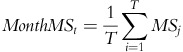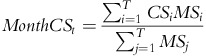Across all asset classes in our study, the monthly correlation surprise series is negatively correlated to the moving average magnitude surprise series. Using these monthly signals, we repeat the conditional returns analysis from Tables 4 and 5 for subsequent one-month holding periods, assuming that positions would only be modified at the end of any given month. The monthly tests cover the same time period as the daily tests presented earlier, and we use the same simple parameters: an 80th percentile threshold for magnitude surprise and a threshold of one for correlation surprise. Tables 7 and 8 show these results for the broad investable indices and short straddles, respectively. The results from this analysis across all three asset universes show that on average, subsequent one-month asset performance is more negative when high magnitude surprise is accompanied by high correlation surprise than when it is accompanied by low correlation surprise. This result is fully consistent with our findings on daily data. As we might expect, many of the annualized return differentials are smaller for monthly data than for daily data, but they are still quite meaningful. Across all tests in Tables 7 and 8, the hit rates (percentage of positive one-month returns) decrease following increases in correlation surprise.Footnote 15

## SUMMARY

We describe how to decompose the Mahalanobis distance (also known as financial turbulence) to derive a measure of correlation surprise across a set of assets. We show, both conceptually and empirically, that our correlation surprise measure is distinct from and incremental to magnitude surprise. Whereas magnitude surprise describes the extent to which asset returns are extreme vis-à-vis their historical distributions, correlation surprise describes the extent to which their interaction is unusual given the historical correlation matrix. Finally, we construct correlation surprise series for three different asset classes and present evidence that the joint occurrence of high magnitude surprise and high correlation surprise foretells higher volatility and lower return than high magnitude surprise in isolation.

These findings have several concrete investment implications. A superior understanding of correlation surprises could enhance trade execution algorithms, which rely on correlation estimates to manage opportunity costs. In addition, risk managers could improve their volatility forecasts by incorporating correlation surprises into their models. And, perhaps most interestingly, portfolio managers may be able to enhance their performance by de-risking when they observe correlation surprises coupled with heightened volatility.